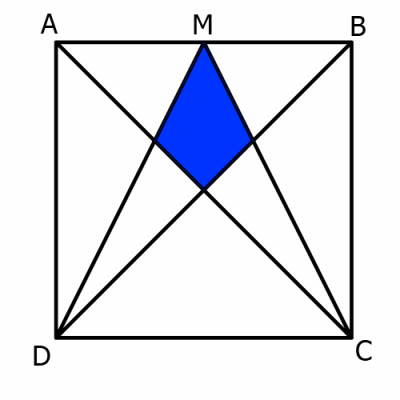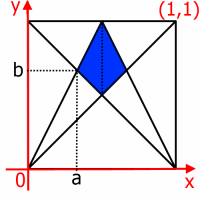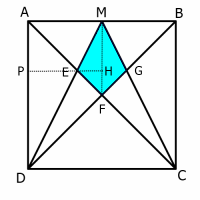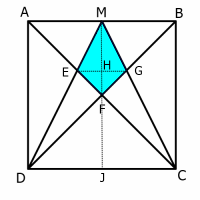#### You may also like### Doodles

Draw a 'doodle' - a closed intersecting curve drawn without taking pencil from paper. What can you prove about the intersections?### Russian Cubes

I want some cubes painted with three blue faces and three red faces. How many different cubes can be painted like that?### Picture Story

Can you see how this picture illustrates the formula for the sum of the first six cube numbers?

# Kite in a Square

##### Age 14 to 16Challenge Level

ABCD is a square. M is the midpoint of the side AB.
By constructing the lines AC, MC, BD and MD, the blue shaded quadrilateral is formed:What fraction of the total area is shaded?

Below are three different methods for finding the shaded area. Unfortunately, the statements have been muddled up. Can you put them in the correct order?

Coordinates(A) The shaded area is made up of two congruent triangles,
one of which has vertices $(\frac{1}{3},\frac{2}{3}), (\frac{1}{2},\frac{1}{2}), (\frac{1}{2},1)$.

(B) The line joining $(0,0)$ to $(\frac{1}{2},1)$ has equation $y=2x$

(C) Area of the triangle $= \frac{1}{2}(\frac{1}{2} \times \frac{1}{6}) = \frac{1}{24}$

(D) The line joining $(0,1)$ to $(1,0)$ has equation $y=1-x$.

(E) Therefore the shaded area is $2 \times \frac{1}{24} = \frac{1}{12}$

(F) The point $(a,b)$ is at the intersection of the lines
$y=2x$ and $y=1-x$.

(G) Consider a unit square drawn on a coordinate grid.

(H) The perpendicular height of the triangle is $\frac{1}{2}-\frac{1}{3}=\frac{1}{6}$.

(I) So $a = \frac{1}{3}, b=\frac{2}{3}$.

(J) The line joining $(0,0)$ to $(1,1)$ has equation $y=x$.

To help you reorder the statements above, here is a set of printable cards for you to cut out.

Similar Figures

(A) As line $AC$ intersects line $MD$ at point $E$,the two opposite angles $\angle MEF$ and $\angle AED$ are equal.

(B) The line $MF$ is half the length of $AD$.

(C) Line $AD$ is parallel to line $MF$, so $\angle EDA$ and $\angle EMF$ are equal, and $\angle EAD$ and $\angle EFM$ are equal (alternate angles).

(D) Therefore, $\triangle AED$ and $\triangle FEM$ are similar.

(E) Therefore, the line $EH$ is half the length of $PE$.

(F) Let ABCD be a unit square.

(G) Therefore, the shaded area $MEFG = \frac{1}{24} \times 2 = \frac{1}{12}$ sq units.

(H) $PH$ has length $\frac{1}{2}$ units, so $PE$ has length $\frac{1}{3}$ units and $EH$ has length $\frac{1}{6}$ units.

(I) $\triangle MEF$ has area $\frac{1}{2}(\frac{1}{2}\times\frac{1}{6}) = \frac{1}{24}$ sq units.

To help you reorder the statements above, here is a set of Printable cards for you to cut out.

Pythagoras(A) The area of $\triangle DMC = 2$ sq units.
The area of $\triangle DFC = 1$ sq unit.
Thus the combined area of $\triangle DFE$, $\triangle CFG$ and
shaded area $MEFG$ is $1$ sq unit.

(B) $(EH)^2+(HF)^2=(EF)^2$
$EH = HF$
$(EH)^2 = \frac{1}{2}(EF)^2$
$EH = \frac{EF}{\sqrt 2}$

(C) Areas of $\triangle DFE$, $\triangle CFG$ and shaded area $MEFG$ are equal
so each must have an area of $\frac{1}{3}$ sq units.

(D) Area of $\triangle MEF = \frac{1}{2}(1 \times EH) = \frac{1}{2}(\frac{EF}{\sqrt 2})$

(E) By Pythagoras, $DF$ has length $\sqrt 2$.

(F) The total area of the square is $4$ sq units, so the shaded area is
$\frac{1}{12}$ the area of the whole square.

(G) Area of $\triangle DFE = \frac{DF \times EF}{2}$
$= \frac{\sqrt 2 \times EF}{2} = \frac{EF}{\sqrt 2}$

(H) So the shaded area $MEFG$ is equal to the area of $\triangle DFE$.

(I) Assume that the sides of the square are each $2$ units long.
Thus, $DJ$ and $FJ$ are each $1$ unit long.

To help you reorder the statements above, here is a set of Printable cards for you to cut out.

Thanks to Jerome Foley for drawing our attention to this problem.